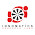## Monday, September 13, 2021

Published September 13, 2021 by with 1 comment

# Why Do We Multiply the Way We Do?

We could just repeatedly add the numbers but we don't. Is the algorithm we use actually faster?

What I'm talking about here is multiplying 219 x 87 like the following:

• 7x9 to get 63
• 7x1 to get 7 and add a 0 to get 70
• 7x2 to get 14 and add two 0's to get 1400
• 8x9 to get 72 and add a 0 to get 720
• 8x1 to get 8 and add two 0's to get 800
• 8x2 to get 16 and add three 0's to get 16000
• add all those together to get 19,053
That's 6 simple multiplications and 6 additions. If we just added 219 to itself 87 times, that's 87 operations so clearly more steps with one big assumption:

you've memorized m x n for all integers m and n from 2 to 10.

This is why we all had to learn times tables. How does this generalize as an algorithm?

Repeated addition is ~ operation per 'smallest of the two numbers', so that is just O(n) where n is the smaller of the numbers.

The algorithm we actually use is a bit harder. It scales as a x b where a and b are the number of digits in the two numbers. How does 'number of digits' scale? That's O(logn) where n is the number. Since it scales as the product of those, that algorithm scales as O(logm * logn) where m and n are the two numbers.

What about the memorized simple multiplications? I have no idea how our memory access scales, but I'm going to just guess it's a constant time operation for simple multiplication so O(1) which doesn't contribute.

For an example with actual calculations, here is the cost of multiplying each number up to 99 by 99 using each algorithm:

It might not be obvious that O(logm * logn) is faster than O(n) but with actual numbers in the plot there it becomes pretty clear.

It's cool to me that a basic math thing we all learn when we're little kids effectively uses a dynamic programming algorithm (memorize all m x n for m and n up to 10; convert every multiplication problem into a combination of m x n problems that you already solved).

#### 1 comment:

1.Thanks for sharing such informative news.
Become Data science expert with Innomatics where you get a great experience and better knowledge.